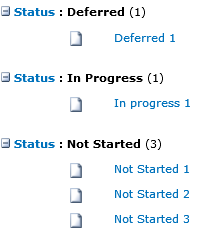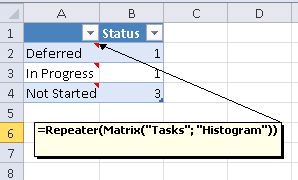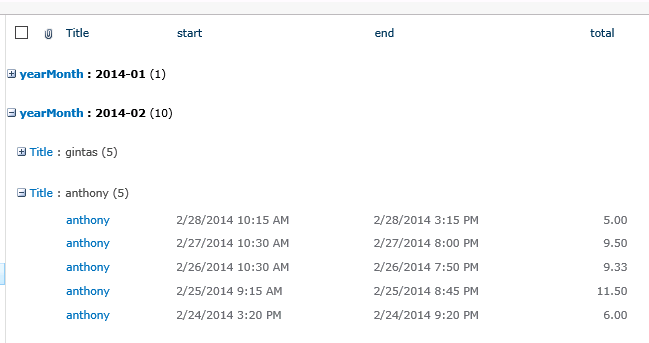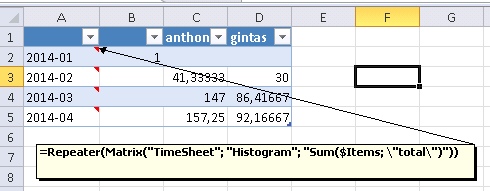# Matrix function

Applies to JungleDocs for SharePoint 2010-2019.

This article describes the formula syntax and usage of the Matrix function in JungleDocs.

## Description

Achieves statistical data transformations using data from grouped lists.

## Syntax

`Matrix(listName; viewName; [formula])`

`Matrix(recordSet; [formula])`

The Matrix function syntax has the following arguments:

• listName   Required. A SharePoint list name.

• viewName   Required. A SharePoint view name.

• formula   Optional. A formula that does calculations with items in a grouped view. If you omit this argument in the Matrix formula, the default function `Count(\$Items)` will be applied to return the number of items. Instead of Count, you can also use the Sum, Average, Median, Min and Max functions (see example).

• recordSet   Required. Any formula that returns a grouped view (e.g., GetView).

## Remarks

• You can also use formulas in Excel sheets to create Word charts.

## Example

### To use Matrix with a grouped view

Let's say you have a grouped view, called Histogram, in the Tasks list.Now, to transfer this view into an Excel sheet, enter the following formula in the Excel template cell :

The result:### To use Matrix with 2-level grouping

The Matrix function also supports 2-level grouping. Let's say you have the Histogram view in the TimeSheet list:in the Excel template, you can use Matrix to display the first level of grouping (year and month) as rows and the second level of grouping (person) as columns. Use this formula:

=Repeater(Matrix("TimeSheet"; "Histogram")

You can also apply further calculations to the data by adding commands to the formula. In the example, we want to Excel to return the sum of grouped items by the total field using the Sum function, so the final formula looks like this:

=Repeater(Matrix("TimeSheet"; "Histogram"; "Sum(\$Items;\"total")"))

The result: